# GATE Questions - OS - CPU Scheduling

Previous GATE questions with solutions on Operating Systems (CPU Scheduling) - CS/IT

GATE-1995
1. Which scheduling policy is most suitable for a time-shared operating systems?
(a) Shortest Job First                        (b) Round Robin
(c) First Come First Server                (d) Elevator

Ans: option (b)
Explanation:
In order to schedule processes fairly, a round-robin scheduler generally employs time-sharing, giving each job a time slot or quantum (its allowance of CPU time), and interrupting the job if it is not completed by then. It is designed especially for time-sharing systems.

GATE-2001
2. Consider a set of n tasks with known runtimes r1, r2, … rn to be run on a uniprocessor machine. Which of the following processor scheduling algorithms will result in the maximum throughput?
(a) Round-Robin         (b) Shortest-Job-First
(c) Highest-Response-Ratio-Next (d) First-Come-First-Served

Ans: option (b)
Explanation:
Throughput means total number of tasks executed per unit time. Shortest Job First has maximum throughput because in this scheduling technique shortest jobs are executed first hence maximum number of tasks are completed.
Note: Highest-Response-Ratio-Next policy favors shorter jobs, but it also limits the waiting time of longer jobs.

GATE-2002
3. Which of the following scheduling algorithms is non-preemptive?
(a) Round-Robin                                       (b) First In First Out
(c) Multilevel Queue Scheduling                (d) Multilevel Queue Scheduling with Feedback

Ans: option (b)

GATE-2006
4. Consider three CPU-intensive processes, which require 10, 20 and 30 time units and arrive at times 0, 2 and 6, respectively. How many context switches are needed if the operating system implements a shortest remaining time first scheduling algorithm? Do not count the context switches at time zero and at the end.
(a) 1    (b) 2    (c) 3    (d) 4

Ans: option(b)
Explanation:
-------------------
PNo    AT      BT
-------------------
A      0       10
B      2       20
C      6       30
-------------------

Time  0: Process A arrives and its the only available process so it runs.
Time  2: Process B arrives, but A has the shortest remaining time (8), so it continues.
Time  6: Process C arrives, but A has the shortest remaining time (2), so it continues.
Time 10: Process A is completed and context switching takes place. B is scheduled as it is the shortest remaining time process.
Time 30: Process B is completed and context switching takes place. Now C is scheduled.

GATE-2007
5. Group 1 contains some CPU scheduling algorithms and Group 2 contains some applications. Match entries in Group 1 to entries in Group 2.
Group I                          Group II
(P) Gang Scheduling              (1) Guaranteed Scheduling
(Q) Rate Monotonic Scheduling    (2) Real-time Scheduling
(R) Fair Share Scheduling        (3) Thread Scheduling
(a) P – 3 Q – 2 R – 1
(b) P – 1 Q – 2 R – 3
(c) P – 2 Q – 3 R – 1
(d) P – 1 Q – 3 R – 2

Ans: option (a)
Explanation:
Gang scheduling is a scheduling algorithm for parallel systems that schedules related threads or processes to run simultaneously on different processors. CLICK TO KNOW MORE
Rate monotonic scheduling is a scheduling algorithm used in real-time operating systems with a static-priority scheduling class. CLICK TO KNOW MORE
Fair Share Scheduling is a scheduling strategy in which the CPU usage is equally distributed among system users or groups, as opposed to equal distribution among processes. It is also known as Guaranteed scheduling.CLICK TO KNOW MORE

GATE-1993
6. Assume that the following jobs are to be executed on a single processor system
-----------------------
Job-Id   CPU-BurstTime
-----------------------
p           4
q           1
r           8
s           1
t           2
-----------------------
The jobs are assumed to have arrived at time  0 and in the order p, q, r, s, t. Calculate the departure time (completion time) for job p if scheduling is round robin with time slice 1.
(a) 4   (b) 10  (c) 11   (d) 12

Ans: option (c)
Explanation:
Execution steps are plotted below
 P Q R S T P R T P R P R 1 2 3 4 5 6 7 8 9 10 11 16

GATE-1995
7. The sequence …………… is an optimal non-preemptive scheduling sequence for the following jobs which leaves the CPU idle for ………………… unit(s) of time.
----------------------------
Job Arrival_Time Burst_Time
----------------------------
1       0.0        9
2       0.6        5
3       1.0        1
----------------------------
(a) {3,2,1),1 (b) (2,1,3},0
(c) {3,2,1),0 (d) {1,2,3},5

Ans: option (a)
Explanation:
Shortest Job First is an optimal solution for non-preemptive scheduling. Hence the sequence should be 3,2,1. For that sequence to occur the CPU should wait for 1 units of time.

GATE-1996
8. Four jobs to be executed on a single processor system arrive at time 0 in the order A, B, C, D. their burst CPU time requirements are 4, 1, 8, 1 time units respectively. The completion time of A under round  robin scheduling with time
slice of one time unit is
(a) 10   (b) 4   (c) 8    (d) 9

Ans: option (d)

GATE-2003
9. A uni-processor computer system only has two processes, both of which alternate 10 ms CPU bursts with 90 ms I/O bursts. Both the processes were created at nearly the same time. The I/O of both processes can proceed in parallel. Which of the following scheduling strategies will result in the least CPU utilization (over a long period of time) for this system?
(a) First come first served scheduling
(b) Shortest remaining time first scheduling
(c) Static priority scheduling with different priorities for the two processes
(d) Round robin scheduling with a time quantum of 5 ms

Ans: option (d)

When Round Robin scheduling is used
We are given that the time slice is 5ms. Consider process P and Q.
Say P utilizes 5ms of CPU and then Q utilizes 5ms of CPU. Hence after 15ms P starts with I/O And after 20ms Q also starts with I/O. Since I/O can be done in parallel, P finishes I\O at 105th ms (15 + 90) and Q finishes its I\O at 110th ms (20 + 90). Therefore we can see that CPU remains idle from 20th to 105th ms.
That is when Round Robin scheduling is used,
Idle time of CPU =  85ms
CPU Utilization = 20/105 = 19.05%

When First Come First Served scheduling scheduling or Shortest Remaining Time First is used
Say P utilizes 10ms of CPU and then starts its I/O. At 11th ms Q starts processing. Q utilizes 10ms of CPU.
P completes its I/O at 100ms (10 + 90)
Q completes its I/O at 110ms (20 + 90)
At 101th ms P again utilizes CPU. Hence,
Idle time of CPU =  80ms
CPU Utilization = 20/100 = 20%

Since only two processes are involved and I\O time is much more than CPU time, "Static priority scheduling with different priorities" for the two processes reduces to FCFS or Shortest remaining time first.
Therefore, Round robin will result in least CPU utilization.

GATE-2004
10. Consider the following set of processes, with the arrival times and the CPU burst times given in milliseconds.
-------------------------------------
Process    Arrival-Time    Burst-Time
-------------------------------------
P1             0             5
P2             1             3
P3             2             3
P4             4             1
-------------------------------------
What is the average turnaround time for these processes with the preemptive shortest remaining processing time first (SROT) algorithm?
(a) 5.50    (b) 5.75     (c) 6.00    (d) 6.25

Ans: option (a)
Explanation:
Execution chart is shown below:
 P1 P2 P4 P3 P1 1 4 5 8 12
Calculate the Turn Around Time (TAT) for each process as shown in the table below.
TAT = Completion Time - Arrival Time
-------------------------------------
Pro    AT      BT   CT     TAT(CT-AT)
-------------------------------------
P1     0       5   12        12
P2     1       3    4         3
P3     2       3    8         6
P4     4       1    5         1
-------------------------------------
Avg TAT = (12+3+6+1)/4 = 5.50

GATE-2006
11. Consider three processes (process id 0, 1, 2 respectively) with compute time bursts 2, 4 and 8 time units. All processes arrive at time zero. Consider the longest remaining time first (LRTF) scheduling algorithm. In LRTF ties are broken by giving priority to the process with the lowest process id. The average turn around time is:
(a) 13 units (b) 14 units            (c) 15 units (d) 16 units

Ans: option (a)
Explanation:
Execution chart is shown below:
 P2 P1 P2 P1 P2 P0 P1 P2 P0 P1 P2 4 5 6 7 8 9 10 11 12 13 14

Calculate the Turn Around Time (TAT) for each process as shown in the table below.
TAT = Completion Time - Arrival Time
-------------------------------------
Pro    AT      BT   CT     TAT(CT-AT)
-------------------------------------
P0     0       2   12        12
P1     0       4   13        13
P2     0       8   14        14
-------------------------------------
Avg TAT = (12+13+14)/3 = 13

GATE-2006
12. Consider three processes, all arriving at time zero, with total execution time of 10, 20 and 30 units, respectively. Each process spends the first 20% of execution time doing I/O, the next 70% of time doing computation, and the last 10% of time doing I/O again. The operating system uses a shortest remaining compute time first scheduling algorithm and schedules a new process either when the running process gets blocked on I/O or when the running process finishes its compute burst. Assume that all I/O operations can be overlapped as much as possible. For what percentage of time does the CPU remain idle?
(a) 0%     (b) 10.6%     (c) 30.0%     (d) 89.4%

Ans: option (b)
Explanation:
--------------------------
Pro    AT   IO   BT    IO
--------------------------
P1     0    2    7     1
P2     0    4   14     2
P3     0    6   21     3
--------------------------
Execution chart is shown below:
 P1 P2 P3 2 9 23 44 47

Total Execution Time = 47
CPU Idle Time = 2 + 3 = 5
Percentage of Idle Time = 5/47 = 10.6%

GATE-2007
13. An operating system uses Shortest Remaining Time first (SRT) process scheduling algorithm. Consider the arrival times and execution times for the following processes: ------------------------------------- Process Execution time Arrival time ------------------------------------- P1 20 0 P2 25 15 P3 10 30 P4 15 45 ------------------------------------- What is the total waiting time for process P2? (a) 5 (b) 15 (c) 40 (d) 55

Ans: option (b)
Explanation:
Execution chart is shown below:
 P1 P2 P3 P2 P4 20 30 40 55 70
Waiting Time = Completion Time - Arrival Time - Execution Time
Waiting Time = 55 - 15 - 25 = 15

GATE-2011
14.  Consider the following table of arrival time and burst time for three processes P0, P1 and P2.
-----------------------------------
Process   Arrival time   Burst Time
-----------------------------------
P0            0 ms          9 ms
P1            1 ms          4 ms
P2            2 ms          9 ms
-----------------------------------
The pre-emptive shortest job first scheduling algorithm is used. Scheduling is carried out only at arrival or completion of processes. What is the average waiting time for the three processes?
(a) 5.0 ms  (b) 4.33 ms  (c) 6.33 ms  (d) 7.33 ms

Ans: option (a)
Explanation:
Execution chart is shown below:
 P0 P1 P0 P2 1 5 13 22
Waiting Time = Completion Time - Arrival Time - Execution Time
-----------------------------------
Pro   AT   BT   CT     WT
-----------------------------------
P0    0    9   13      4
P1    1    4    5      0
P2    2    9   22     11
-----------------------------------
Average Waiting Time = (4+0+11)/3 = 5ms

GATE-2012
15.  Consider the 3 processes, P1, P2 and P3 shown in the table
----------------------------------------------
Process     Arrival time    Time unit required
----------------------------------------------
P1              0                    5
P2              1                    7
P3              3                    4
----------------------------------------------
The completion order of the 3 processes under the policies FCFS and RRS (round robin scheduling with CPU quantum of 2 time units) are
(a) FCFS: P1, P2, P3 RR2: P1, P2, P3
(b) FCFS: P1, P3, P2 RR2: P1, P3, P2
(c) FCFS: P1, P2, P3 RR2: P1, P3, P2
(d) FCFS: P1, P3, P2 RR2: P1, P2, P3

Ans: option (c)
For FCFS, the order of execution will be according to their arrival time. The one which arrives first will be scheduled first. So the sequence of completion according to the FCFS policy will be P1, P2, P3. Since we have such option only in option-c its the right answer. Then what about RRS policy. Is the completion order given in option-c correct? Yes it is!
The execution chart and ready queue sequence at each time unit is given below. The orange box shows the Ready Queue Sequence and Blue shows the Execution Sequence. If the arrival time differs for the processes, always try to draw the ready queue also. Ready Queue will give you the the exact process to be scheduled next.

1.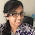hi,

1.2.can anyone please explain execution chart of ques 12??

2.awesome work man !!
Thanks

3.Great work yaar...
Thank you....

4.So nice of u...thank u

5.thanx buddy ...it was helpful :)

6.good job buddy

7.8.9.10.GREAT!!! thnks.

11.12.Very nice set of questions man ... thanks

13.great work....

14.thnxs buddy :)

15.16.so nice of u. thank u.....

17.great work...thnks a lot..!!

18.In last question for RRS, process completion must be P3,P1,P2 . If not please explain. And please explain question 11 more clearly.

1.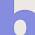Check explanation

2.m appreciate with u...
the last ques ans is wrong...
that must be p3,p1,p2..
btw thnx for giving all those ques ans with explanation..

19.for question 15, options do not seem to be correct. The RR scheduling would execute three processes like P1,P2,P3,P1,P2,P3,P1,P2,P2....Can any one please confirm the same or correct m eif i am wrong..

1.Check explanation. Option c is correct

2.but how...??
when i solved it in my copy ..
m get p3,p1,p2

3.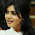Yes..I'm also getting P3,P1,P2 ..at time 4,P3 already arrived so we can schedule it right..?
Btw thanku for the questions.. great work :)

20.Answer for question-9 would be (d)

1.Thank you for the correction.

21.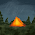good work!!!!

22.thanks............

23.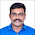24.can u pls post for paging problems

25.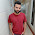what is service time in scheduling algorithm.

26.Thank You brother! Really helped a lot!

27.28.29.This comment has been removed by the author.

30.31.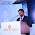Answer for question no 15 is correct. Because at time 2 when P1 is pre-emptied it goes to ready queue directly. At this time P3 has not arrived. So P1 gets its second chance before P3 gets its first chance. Note when P3 arrived P1 was already in queue waiting for its next chance. So the order of execution goes like P1, P2, P1, P3, ... This is well explained by the author using ready queue.

32.33.34.